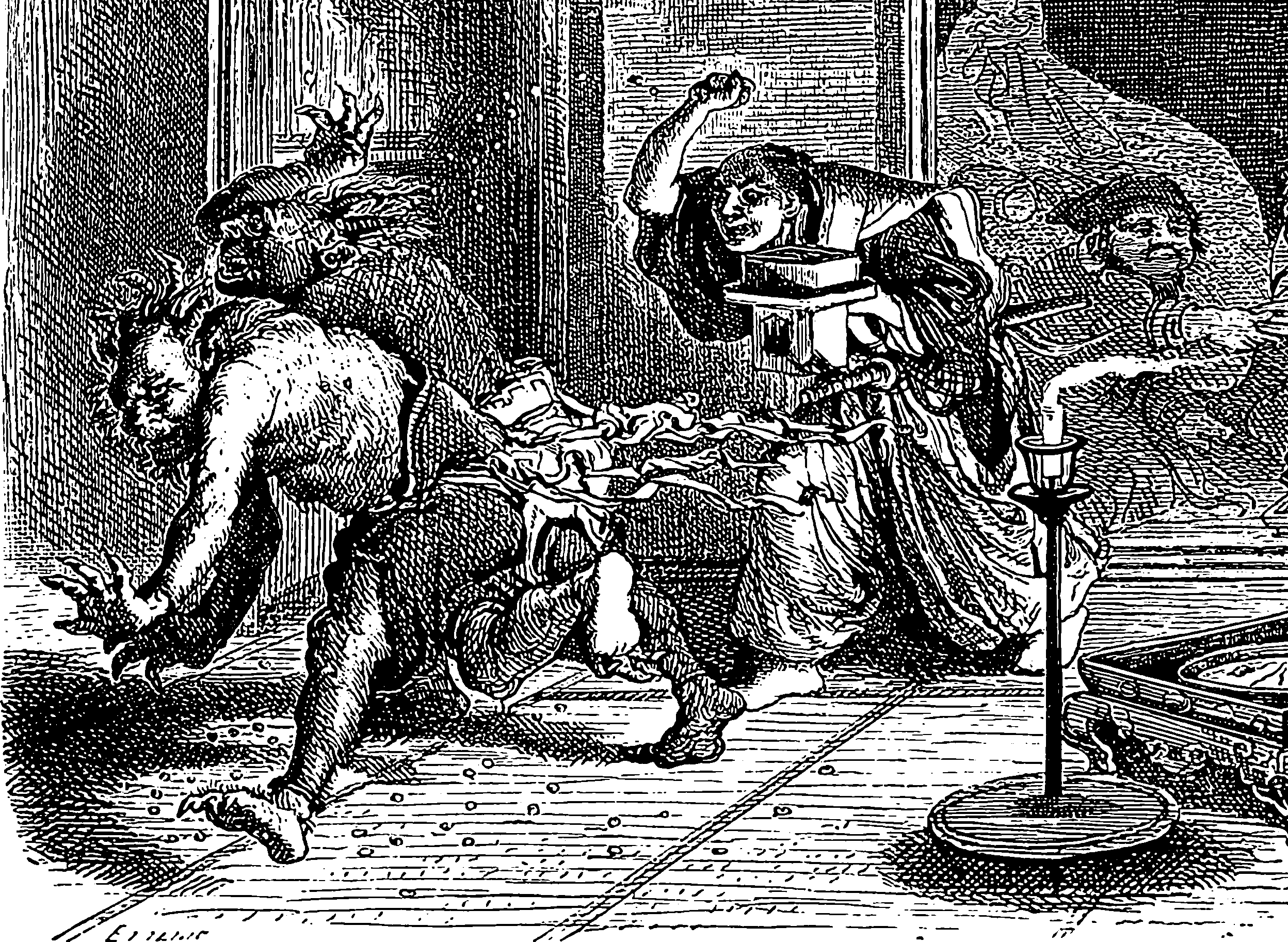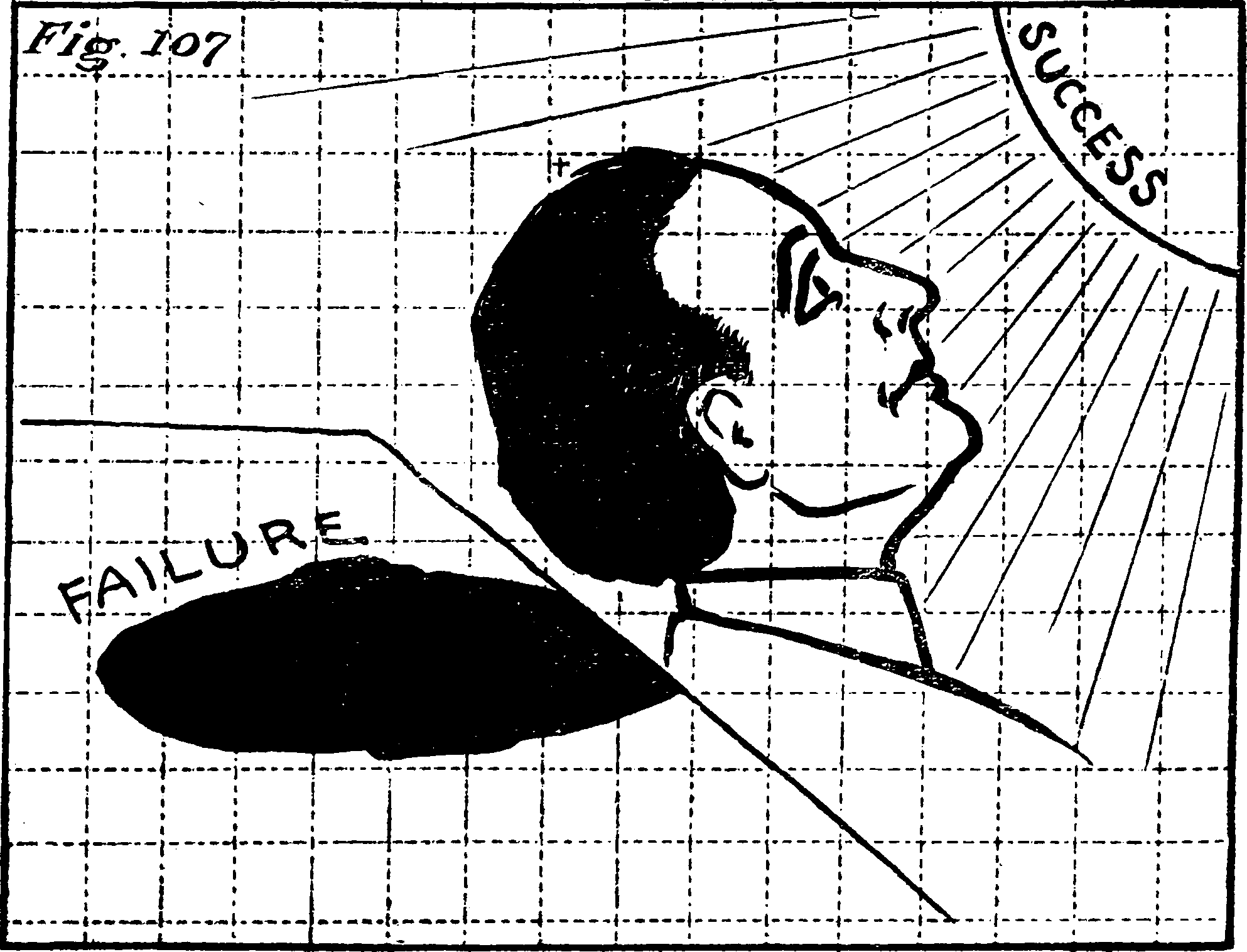# Bounded rationality

## Plus miscellaneous rationality postulates restricting von Neumann-MorgensternProspect theory, Kahnemann-and-Tversky style. Rational Heuristics, Gigerenzer-and-Todd style. Docility, Herbert-Simon-wise. Behavioural economics more generally.Ruben Bolling## For risk

see risk perception.

## For learning and action

Can we get anywhere with predictive coding?

## …and trust

Maybe trust machines.

## In groups

Let us think of the wisdom of crowds.

## Incoming

Thinking about this very computationally is the mainstay of APXHARD, e.g. P ⊂ NP causes Political Polarization

• Reduce Noise, Not Cognitive Biases

• How To Reduce Decision Noise

• DRMacIver’s Notebook: Three key problems with Von-Neumann Morgenstern Utility Theory

The first part is about physical difficulties with measurement—you can only know the probabilities up to some finite precision. VNM theory handwaves this away by saying that the probabilities are perfectly known, but this doesn’t help you because that just moves the problem to be a computational one, and requires you to be able to solve the halting problem. e.g. choose between $$L_1=p B+(1-p) W$$ and $$L_2=q B+(1-q) W$$ where $$p=0.0 \ldots$$ until machine $$M 1$$ halts and 1 after and $$q$$ is the same but for machine $$M 2$$.

The second demonstrates that what you get out of the VNM theorem is not a utility function. It is an algorithm that produces a sequence converging to a utility function, and you cannot recreate even the original decision procedure from that sequence without being able to take the limit (which requires running an infinite computation, again giving you the ability to solve the halting problem) near the boundary.

### No comments yet. Why not leave one?

GitHub-flavored Markdown & a sane subset of HTML is supported.